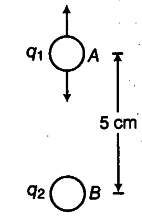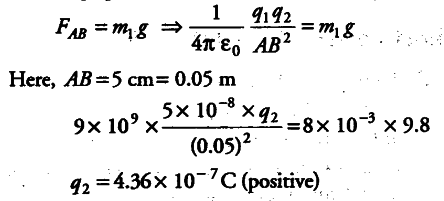# A free pith-ball of 8 g carries a positive charge of 5 x \${{10}^{-8}}\$C

A free pith-ball of 8 g carries a positive charge of 5 x \${{10}^{-8}}\$C. What must be the nature and magnitude of charge that should be given to a second pith-ball fixed 5 cm vertically below the former pith-ball so that the upper pith-ball is stationary?

Here, charge on the pith-ball A, { q }_{ 1 } = 5 x {{10}^{-8}} C
Mass of the pith-ball A, { m }_{ 1 } = 8g = 8 x {{10}^{-3}} Kg
The weight { m }_{ 1 } g or the pith-ball A acts vertically downwards.Let { q }_{ 2 } be charge on the pith-ball B held 5 cm below the pith ball A so that the pith-ball A remains stationary.
It can be possible only, if the charges on two pith-balls are of same signs, i.e. if charge on the pith-ball A is positive, the charge on B should also be positive. As such the force on the pith-ball A due to.B, i.e. FM will act vertically upwards.
For charge { q }_{ 1 } to remain stationary,